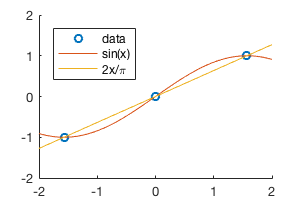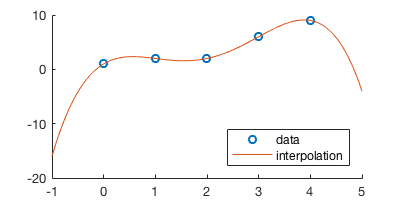# Lecture 8: Interpolation¶

Date: 09/26/2017, Tuessday

In :

format compact


## A simple example¶

Make 3 data points

In :

xp = [-pi/2, 0, pi/2];
yp = [-1, 0, 1];


The two functions below both go through the 3 data points.

In :

f = @(x) sin(x);
g = @(x) 2*x/pi;


There are ways to plot a function symbolically/analytically (for example), but those methods have a lot of limitations and you don’t have detailed controls on them.

So we stick to the most standard way of plotting: evaluate the function value on a lot of points to make the line look smooth.

In :

x = linspace(-2,2,1e3); % a 1000 grid points from -2 to 2


Always remember to surpress the output (by ;) when defining this kind of large array. MATLAB WILL print all the elements in an array/matrix no matter how large it is. Sometimes your program will die just because it wants to print $$10^{10}$$ numbers.

Now we can plot the functions and data points.

In :

%plot --size 300,200
hold on
plot(xp,yp,'o')
plot(x,f(x))
plot(x,g(x))
legend('data','sin(x)','2x/\pi','Location','northwest')## Polynomial interpolation¶

In :

% make some data points
n = 5;
px = [0 1 2 3 4];
py = [1 2 2 6 9];


n data point can be precisely fitted by a n-1 degree polynomial $$p=c_1+c_2x+...+c_nx^{n-1}$$.

The coffeicients $$c=[c_1,c_2,...c_n]^T$$ satisfy the equation

$Vc=y$

where $$y=[y_1,y_2,...,y_n]^T$$ is the data points you want to fit, and V is the vandermode matrix only containing powers of $$x_k$$, the data points.

In :

% calculate the vander matrix by loop
V = zeros(n);
for j=1:5
V(:,j) = px.^(j-1);
end
V

V =
1     0     0     0     0
1     1     1     1     1
1     2     4     8    16
1     3     9    27    81
1     4    16    64   256


The built-in vander is flipped left-right. Both forms are correct as long as your algorithm is consistent with the matrix.

In :

vander(px)

ans =
0     0     0     0     1
1     1     1     1     1
16     8     4     2     1
81    27     9     3     1
256    64    16     4     1


Now we can solve $$Vc=y$$ by $$c=V^{-1}y$$. In MATLAB backslash form it is c=V\y. Note that the actual code never computes $$V^{-1}$$, but use something like LU factorization/Gaussian elimination to solve the system. (it is almost always a bad idea to compute the inverse of a matrix). But thinking about $$V^{-1}y$$ helps you to remember the order of V and y in the command (e.g. is it V\y or y\V ?).

The code below throws an error because y is a row vector.

In :

c = V\py

Error using \
Matrix dimensions must agree.



You need a column vector on the right side, just like how you write the equation mathematically.

In :

c = V\py'

c =
1.0000
5.6667
-7.5833
3.3333
-0.4167


Now we have the coefficients $$c$$, we can write the polynomial $$p=c_1+c_2x+...+c_nx^{n-1}$$ as a MATLAB function.

Here’s a naive way to evaluate the polynomial. You should write a loop instead. Also consider Horner’s method to achieve optimal performance.

In :

my_ploy = @(c,x) c(1) + c(2)*x + c(3)*x.^2 + c(4)*x.^3 + c(5)*x.^4 ;

In :

% evaluate the function on a lot of data points
xf = linspace(-1,5,100);
yf = my_ploy(c,xf);

In :

%plot --size 400,200
hold on
plot(px,py,'o')
plot(xf,yf)
legend('data','interpolation','Location','southeast')